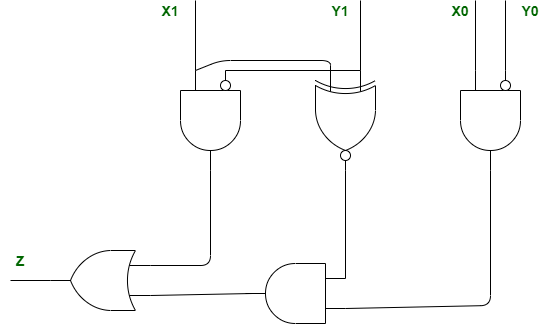GeeksforGeeks App
Open AppBrowser
Continue

# ISRO | ISRO CS 2020 | Question 3

The following circuit compares two 2-bit binary numbers, X and Y represented by X1X0 and Y1Y0 respectively. (X0 and Y0 represent Least Significant Bits)Under what condition Z will be 1 ?
(A) X > Y
(B) X < Y

(C) X = Y
(D) X! = Y

`Z = X1Y1' + (X1⊙Y1)X0Y0'`